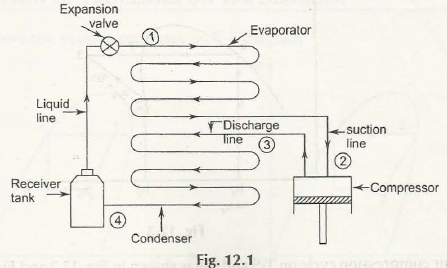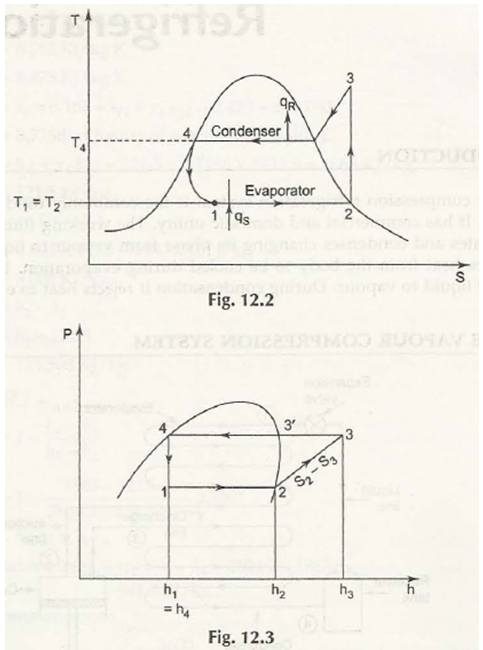Figure above 12.1 shows a simple vapour compression refrigeration system which includes

• compression
• Expansion
• Vaporization
• condensation

(a)  Compression: The vapour at low temperature and low pressure i.e. to (point 2) enters into compressor where it is fully compressed reversible and adiabatically (isentropic ally). There is an increase of pressure and temperature which passes through discharge line i.e. (point 3).

(b)  Condensation: The vapour at high temperature and pressure enters into condenser where it is fully condensed to high pressure liquid i.e. to (point 4) which is collected by tank which is the main receiver.

(c)   Expansion: The liquid which is from receiver tank easily passes through the valve which is in extension in nature where it is throttled (isoenthalpy) and passes through at rate which is controlled in nature  after reducing temperature and pressure.

(d)  Vaporisation: In evaporation the liquid which is at low pressure and temperature easilyevaporates and converts into vapour. During vaporization process, the liquid is easily absorbs by latent heat of vaporfrom the medium (air, water or brine) which needs to be cooled.Vapour compression cycle on T-S and P-h is shown in Fig. 12.2 and Fig. 12.3 respectively.

12.2.1 Coefficient -of Performance of Refrigerator for Vapour Compression Cycle

Coefficient of Performance of refrigerator for vapour compression cycle is stated as

COP = h2 – h1 / h3 – h2 ( COP = heat rejected evaporator / work input to compressor)

2.2.2 The Quantity of Refrigerant (m r) Circulated in the Refrigeration Plant

The Quantity of refrigerant is stated as

m r = 210 T / h2 – h1 kg / min

where T = Load in tons of refrigeration on the refrigerator

12.2.3 Power Required to Drive the Compressor

Power = m r (h3 – h2) / 60 KW

Here  h is in KJ / kg

12.2.4 Quantity of Cooling Water (me) Circulated in the Condenser

Quantity of cooling water which is circulated in the condenser per minute can be easily found out from the

equation m, Cc (t0 – t;) = m, (h3 – h4).

where

mc = mass of cooling water circulated per minute

Cc = specific heat of cooling water

t0 =outlet temperature of cooling water

t1 = inlet temperature of cooling water.

Links of Previous Main Topic:-

Links of Next Mechanical Engineering Topics:-

### Submit Your Assignment### Customer Reviews

My Homework Help
Rated 5.0 out of 5 based on 510 customer reviews at